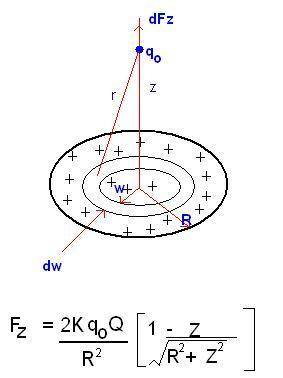# How To apply Binomial Therem For, Z = 0 and Z R

• urduworld

#### urduworldHi Friends Please Solve my problem

dq = Surface Charge
da = Surface Area
qo = Point Charge
Distance from Center of Ring to Point Charge = Z

I want to know how I ca apply binomial theorem to solve the equation
If
Z = 0
Z >> 0 (Very greater)